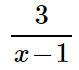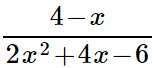### Day 51 - Rational Expressions - 10.29.14

 UpdatesAverage on the Quadratics Test (Multiple Choice) = 85%Remediation/Retest ProceduresBell RingerRational ExpressionsWhat is a rational number?a number that is represented by an integer divided by another integera number that is a ratio of two integersa number that is a fraction of two integersall of the abovenone of the aboveWhich number(s) are not allowed to divide by?01-1none of the aboveWhich number would cause the following expression to be undefined?013none of the aboveWhich number would cause the following expression to be undefined?41-31, -3none of the aboveReviewPrerequisitesDividing by 0Rational NumbersNumerator and DenominatorPolynomialsLessonRational FunctionsPolynomial / PolynomialExcluded ValuesGraphs at Excluded ValuesCheckpointsExit TicketPosted on board at end of block. Lesson Objective(s)How can rational expressions be simplified?How are excluded values identified in rational expressions?Standard(s)Solving Rational Equations - 12.9CC.9-12.A.CED.1 Create equations that describe numbers or relationship. Create equations and inequalities in one variable and use them to solve problems. Include equations arising from linear and quadratic functions, and simple rational and exponential functions.CC.9-12.A.REI.11 Represent and solve equations and inequalities graphically. Explain why the x-coordinates of the points where the graphs of the equations y = f(x) and y = g(x) intersect are the solutions of the equation f(x) = g(x); find the solutions approximately, e.g., using technology to graph the functions, make tables of values, or find successive approximations. Include cases where f(x) and/or g(x) are linear, polynomial, rational, absolute value, exponential, and logarithmic functions.CC.9-12.A.REI.2 Understand solving equations as a process of reasoning and explain the reasoning. Solve simple rational and radical equations in one variable, and give examples showing how extraneous solutions may arise.Dividing Polynomials - 12.5CC.9-12.A.APR.6 Rewrite rational expressions. Rewrite simple rational expressions in different forms; write a(x)/b(x) in the form q(x) + r(x)/b(x), where a(x), b(x), q(x), and r(x) are polynomials with the degree of r(x) less than the degree of b(x), using inspection, long division, or, for the more complicated examples, a computer algebra system.Mathematical Practice(s)#1 - Make sense of problems and persevere in solving them#2 - Reason abstractly and quantitatively#4 - Model with mathematics#5 - Use appropriate tools strategically#7 - Look for and make use of structurePast CheckpointsN/A